# Molecular Orbital Theory and Hydrogen Bonding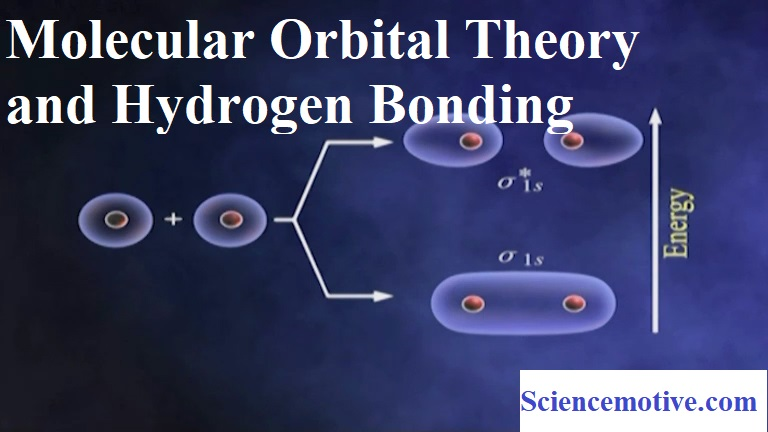Molecular Orbital Theory and Hydrogen Bonding

Molecular Orbital Theory and Hydrogen Bonding:

Molecular Orbital Theory: This theory was developed by F.Hund and R.S Mulliken. The important postulates of this theory are:
(i) When two atomic orbitals combine or overlap, they lose their identity and form new orbitals. The new orbitals formed are called molecular orbitals.
(ii) Only those atomic orbitals can combine to form molecular orbitals which have comparable energies and proper orientations.
(iii) The number of molecular orbitals formed is equal to the number of combining atomic orbitals.
(iv) When two atomic orbitals combine, they form two new orbitals called ‘bonding molecular orbital’ and ‘antibonding molecular orbital’.
(v) The bonding molecular orbital (BMO) has lower energy and hence greater stability than the corresponding antibonding molecular orbital (ABMO).
(vi) The bonding molecular orbitals are represented by σ (sigma), π (Pi), δ (delta), and antibonding are represented by σ*, π*, δ*.
(vii) The shapes of the molecular orbitals formed depend upon the type of combining atomic orbitals.
(viii) The molecular orbitals are filled according to 3 rules – Aufbau principle, Pauli’s exclusion principle, and Hund’s rule.

Formation of molecular orbitals – Linear Combination of Atomic Orbitals (LCAO) method:
Molecular orbitals are formed by the combination of atomic orbitals by an approximate method known as the Linear Combination of Atomic Orbitals (LCAO). According to this theory, the combinations of atomic orbitals take place by the addition and subtraction of wave functions of atomic orbitals. The M.O formed by the addition of A.Os is called the bonding molecular orbital (BMO) and by the subtraction of A.Os is called the anti-bonding molecular orbitals (ABMO).
Bonding Molecular Orbitals                              Anti Bonding Molecular Orbitals
Formed by the addition of atomic orbitals            Formed by the subtraction of atomic orbitals
Lower energy, greater stability                             Higher energy, lower stability
Represented by σ and π                                        Represented by σ* and π*.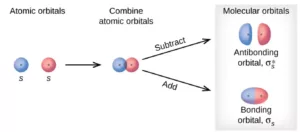Conditions for combination to atomic orbitals to form molecular orbitals:
(i) The combining atomic orbitals should have comparable energy.
(ii) The combining atomic orbitals must have the proper orientation.
(iii) The extent of overlapping should be large.

Types of Molecular Orbitals:
(i) M.Os of diatomic molecules are designated as σ (sigma), π (Pi), δ (delta), etc. the σ (sigma) M.Os are symmetrical about the bond axis, while the pi M.Os are not symmetrical.
(ii) Linear combination of two 1s atomic orbitals produces two M.Os – a BMO σ1s and an ABMO σ*1s.
(iii) Similarly linear combination of two 2s atomic orbitals produces two M.Os – σ2s and σ*2s.
(iv) If the z-axis is taken as the internuclear axis, a linear combination of two 2pz orbitals produces two sigma M.Os σ2pz and σ*2pz.
(v) While the combination of 2px and 2py orbitals produce MO’s which are not symmetrical about the bond axis. So two 2px orbitals produces 2 sigma MO’s – π2px and π*2px and two 2py orbitals produce 2 M.Os – π2py and π*2py.

Energies of various Molecular Orbitals:
The various M.Os are filled in the increasing order of their energies (Aufbau Principle). The increasing order of energy is:
σ1s < σ*1s < σ2s < σ*2s < (π2px = π2py) <σ2pz < (π*2px= π*2py) < σ*2pz
For O2, F2 and Ne2, the order is:
σ1s < σ*1s < σ2s < σ*2s < σ2pz < (π2px = π2py) < (π*2px= π*2py) < σ*2pz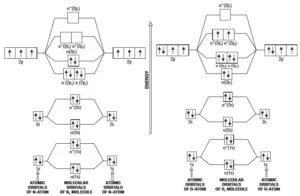Molecular Orbital Diagram for N2              Molecular Orbital Diagram for O2
Bond Order: It is defined as the half of the difference between the number of bonding electrons (Nb) and the number of anti-bonding electrons (Na).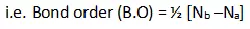A molecule is stable only if the bond order is positive. (i.e. Nb >Na). a negative bond order (i.e. Nb < Na or Nb = Na) means an unstable molecule.
For a single bond, B.O = 1, for a double bond B.O = 2, and so on. The bond order gives an approximate measure of the bond length. In general, as the bond order increases, bond length decreases, and bond enthalpy increases.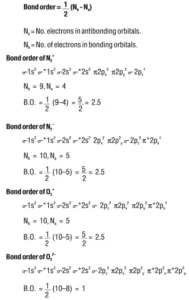Hydrogen Bonding: The Bond which is formed between hydrogen and electronegative species such as N, O, and F. It is weaker than a covalent bond but stronger than van der Waal’s force. It is represented by the dotted line (………).
Reason for Hydrogen Bonding: When H is bonded to a strongly electronegative atom X, the shared electron pair is shifted more towards X. So, the H atom gets a slightly positive charge (δ+) and the electronegative atom gets a slight negative charge (δ). This results in the formation of a polar molecule. The electrostatic force of attraction between these polar molecules is termed H-bonding.
Thus, the hydrogen bond between the hydrogen atom bonded to the electronegative atom X may be represented as follows:
– – – X – H – – – X – H – – – X – H – – –
In such a situation, the hydrogen atom is attached simultaneously to two electronegative atoms. Hence it acts as a bridge between the two and is, therefore, called the hydrogen bridge.

Types of Hydrogen Bonding: There are two types of H bonds- intermolecular H-bonding and intramolecular H-bonding.
(i) Inter-molecular H bonding: It is the H bond formed by the H atom of one molecule and the electronegative atom of another molecule.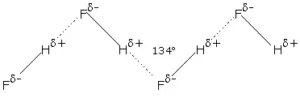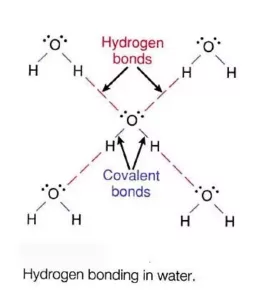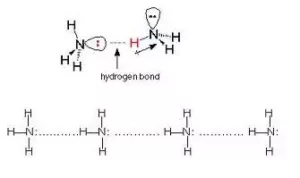Intermolecular H bonding influences the physical properties of the compounds. For example, water (H2O) is a liquid with a high boiling point but hydrogen sulphide (H2S) is a gas. This is because in water intermolecular H bonding is possible which is not possible in H2S.

(ii) Intra-molecular Hydrogen Bonding: It is the H bond formed between the H atom and the electronegative atom of the same molecule.
An important example of intramolecular hydrogen bonding is that of o–nitrophenol. The boiling point of o–nitrophenol is 214 0C as compared to 279 0C for p–nitrophenol. Further o–nitrophenol is more volatile in steam and less soluble in water than the other two isomers. All the above facts can be explained on the assumption that o–nitrophenol contains hydrogen bond represented as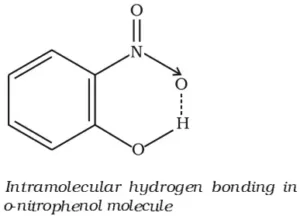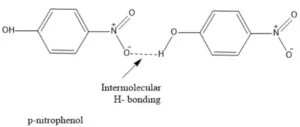Due to the intramolecular hydrogen bonding, the solubility of o–nitrophenol in water is also reduced. In m– and p– isomers, intramolecular hydrogen bonding is not possible so intermolecular hydrogen bonding takes place. This explains higher boiling points of m– and p–isomers.
Conditions for Hydrogen Bonding: In the case of intermolecular hydrogen bonding, the most important condition is that the molecules must contain one hydrogen atom linked to one highly electronegative atom. In the case of intramolecular hydrogen bonding, the following conditions are favorable for hydrogen bonding:
(i) The molecule should contain two groups such that one group contains an H–atom linked to a high electronegative atom and the other group should also contain a highly electronegative atom linked to a lesser electronegative atom.
(ii) The molecule should be planar.

Importance of Hydrogen Bond:
(i) Hydrogen Bonds are important in fixing properties such as solubilities, melting points, and boiling points and in determining the form and stability of crystalline structures.
(ii) Hydrogen bonds are important in biological systems. Proteins, for example, contain both CO and NH groups and hydrogen bonds can be formed to bridge the space between H and O. The structure and hence the properties of proteins depend upon the existence of hydrogen bonds.
(iii) Hydrogen bonding within a single molecule is one of the chief factors in determining the structure of important biological substances.

Molecular Orbital Theory and Hydrogen Bonding